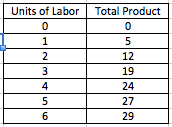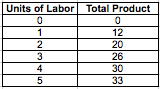# AP Microeconomics : AP Microeconomics

## Example Questions

1 2 5 6 7 8 9 10 11 13 Next →

### Example Question #92 : Competition

Patents, limiting the number of licenses available, and economies of scale are all examples of:

None of the other answers are correct.

barriers to entry

factors of production

sources of demand

sources of supply

barriers to entry

Explanation:

Patents, limiting the number of licenses available, and economies of scale can all hinder a firm's ability to enter the market. A patent prevents a firm from replicating a product that originated from another firm. A limited number of licenses can exclude firms who are unable to obtain licenses from entering the market. Economies of scale can prevent smaller firms from entering the market by making such an action cost-prohibitive.

### Example Question #91 : Perfectly Competitive Markets

Labor, capital, human capital, and natural resources are all examples of:

factors of production

None of the other answers are correct.

sources of demand

barriers to entry

sources of supply

factors of production

Explanation:

All of these are examples of factors of production, which are the inputs necessary for a production process.

### Example Question #93 : Competition

A consumer's indifference curves for two different goods will be a straight lines when:

The prices of the two goods are equal

Utility is maximized

Both goods are perfect substitutes

None of the other answers are correct.

Both goods are perfect compliments

Both goods are perfect substitutes

Explanation:

When both goods are perfect substitutes, the consumer is indifferent between the amount of consumption of the two goods. Thus, his/her indifference curves will be straight lines, because the rate of substitution between the two goods will be constant.

### Example Question #94 : Competition

Which of the following market structures is characterized by low barriers to entry, homogeneous products, and a large number of firms?

Oligopoly

Perfect Competition

Monopolistic Competition

Monopoly

Perfect Competition

Explanation:

In perfectly competitive markets, there are low barriers to entry (think of a food truck--all one needs in order to operate are a truck, fuel, cooking equipment, and a business license), homoegenous products (consider a farm--nearly all cucumbers are identical to one another), and a large number of firms (this is what makes the environment so competitive).

In monopolistic competition and oligopolies, products tend to be heterogeneous, and in a monopoly, there are extremely high barriers to entry.

### Example Question #123 : Ap Microeconomics

For which of the following market structures is demand always equal to marginal revenue?

Oligopoly

Monopoly

Perfect competition

Monopolistic competition

Perfect competition

Explanation:

The marginal revenue curve always has the same intercept on the price axis as the demand curve and twice the slope of the demand curve.

For market structures of oligopoly, monopoly, and monopolistic competition, the firm faces a demand curve that is downward sloping (indicated market power), so the marginal revenue curve would be steeper than the demand curve.

For perfectly competitive market structures, however, the firm faces a demand curve that is horizontal, with a slope of 0 (i.e. it is perfectly elastic). For a firm in perfect competitive market structure, therefore, the marginal revenue curve, which has the same intercept and twice the slope, results in the exact same curve as the demand curve.

### Example Question #1 : Marginal Revenue Product Of Labor Mrp

The following question will refer to this table:If the wage paid to labor is $8 and the price of each unit of output is$2, how many units of labor will be hired?

one

five

four

two

three

four

Explanation:

A firm will hire labor until the marginal revenue product of labor is lower than the cost of labor/wage, which is $8. The marginal revenue product of labor is the marginal product of labor multiplied by the product's price. The marginal revenue of the fourth unit of labor is$10 (five units multiplied by $2) and the marginal revenue of the fifth unit of labor is$6 (three units multiplied by $2). Thus, the firm will hire four units of labor. ### Example Question #2 : Marginal Revenue Product Of Labor Mrp A firm is hiring labor in a perfectly competitive labor market. If the marginal revenue product of labor is greater than the wage rate, which of the following is true? Possible Answers: The firm should invest in more capital. The firm should hire more labor. The firm is maximizing its profit. The firm is losing money. None of the other answers. Correct answer: The firm should hire more labor. Explanation: A profit maximizing firm will hire labor until the marginal product of labor is greater than the wage rate. If the marginal product of labor is greater than the wage rate, then the firm should hire more labor until the two values are equal. ### Example Question #3 : Marginal Revenue Product Of Labor Mrp In a perfectly competitive labor market, an employer will continue hiring until ___________ . Possible Answers: wage equals marginal product of labor. wage equals the price of the good or service produced. wage equals marginal cost of labor. wage equals marginal revenue. wage equals marginal revenue product of labor. Correct answer: wage equals marginal revenue product of labor. Explanation: Like any market, the profit maximization level is the point at which marginal cost equals marginal revenue. In this case, the marginal cost of labor is the labor wage. Thus, the profit maximization point for the employer is the point at which wage equals the marginal revenue product of labor. ### Example Question #4 : Marginal Revenue Product Of Labor Mrp Refer to the following chart for this question:If each product is sold for$2, what is the marginal revenue product of labor for the fourth unit of labor?

$4$8

$10$12

$6 Correct answer:$8

Explanation:

The marginal revenue product of labor for the fourth unit of labor is its marginal product multiplied by the cost of the product. The marginal product of the fourth unit of labor is 4 (the difference between total production at four units of labor and three units of labor), and cost of the product is $2, so the marginal revenue product of labor for the fourth unit is$8.

### Example Question #5 : Marginal Revenue Product Of Labor Mrp

Refer to the following chart for this question:If each product is sold for $2, and each unit of labor costs$10, how many units of labor will the firm hire?

one

three

five

two

four